# SBI PO Reasoning Questions 2019 (Day-04) High Level-New Pattern

SBI PO 2019 Notification is about to come and it is the most awaited exam among the aspirants. We all know that new pattern questions are introducing every year in the SBI PO exam. Further, the questions are getting tougher and beyond the level of the candidate’s expectations.

Our IBPS Guide is providing High-Level New Pattern Reasoning Ability Questions for SBI PO 2019 so the aspirants can practice it on a daily basis. These questions are framed by our skilled experts after understanding your needs thoroughly. Aspirants can practice these high-level questions daily to familiarize with the exact exam pattern. We wish that your rigorous preparation leads you to a successful target of becoming SBI PO.

[WpProQuiz 4653]

## Click Here for SBI PO Pre 2019 High-Quality Mocks Exactly on SBI Standard

Directions (1-4): Study the following information and answer the questions given below.

Mr. Dan Brown has applied for an entrance exam and the following points are known about his roll number which is an eight digit number.

Note:The roll number doesn’t starts with zero. Zero is not considered as an even digit at any instance. Also, zero doesn’t come more than once in the roll number.

(i) The Two digit number formed by 3rd and 4th digits respectively is square of the number formed by 5th and 6th digits.

(ii) 8th and 1st digits are an even numbers.

(iii)The Number formed by 7th and 8th digits is a product of two consecutive natural numbers.

(iv) The Two digit number formed by 4th and 7th digits respectively is not a perfect square.

(v) Sum of the two-digit numbers formed by 7th and 8th digits, and 1st and 2nd digits is a product of two consecutive natural numbers.

(vi)The Numbers formed by 7th and 8th digits is six less than the number formed by 1st and 2nd digit.

(vii) In the number, three digits have been used two times while other two digits have been used only once.

1) What is the sum of the digits of Mr. Dan Brown’s roll number?

a) 28

b) 32

c) 30

d) 34

e) None of these

2) Which of the following is the sixth digit in the roll number?

a) 8

b) 3

c) 4

d) 5

e) 2

3) How many odd digits are there in the even numbered positions and how many even digits are there in the odd numbered positions respectively?

a) Three – Two

b) Two – Three

c) Four – Two

d) Four – Four

e) None of these

4) What is the product of the digits which are at the prime numbered positions?

a) 249

b) 160

c) 0

d) 320

e) None of these

Directions (5-8): Study the following information and answer the questions given below.

There are nine countries – Africa (A), Bangladesh (B), China (C), Denmark (D), Egypt (E), Fiji (F), Greece (G), Hungary (H) and Italy (I) which are neighbour to each other as shown in the fig. below.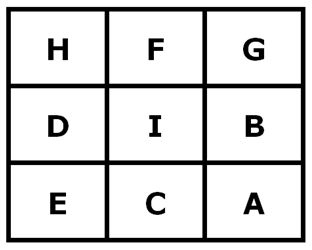Note: H has three neighbouring countries (D, I and F); C has 5 neighbouring countries (D, I, B, E and A) and I has eight neighbouring countries (H, F, G, B, D, E, C and A) and so on.

A, B, C, D, E, F, G, H and I are nine airlines belong to nine different countries which are mentioned above. Each airlines belonging to a country goes to one of its neighbouring country. Not more than one airline goes to the same country. All the information is not necessary to be in the same order.

Also it is known that,

(i) Airline-B goes to Country E

(ii) Country H receives airline from Country F

(iii) Airline-H belongs to Country A

(iv) Only one Airline whose name and the country it belongs to are same.

(v) Airline-D belongs to Country H

(vi) Airline-I belongs to Country C

(vii) Airline from Country G goes to Country F

(viii) Airline-F goes to Country I

(ix) Airline from Country B goes to Country G

(x) Only one Airline whose name and the country it goes to are same.

(xi) Airline-E goes to Country A

5) Which of the following Country does Airline-D goes to?

a) Country H

b) Country F

c) Country D

d) Country C

e) Country E

6) Which of the following Airline goes to Country B?

a) Airline-A

b) Airline-I

c) Airline-C

d) Airline-D

e) None of these

7) Which of the following statement is true?

I) Airline-C belongs to Country B and goes to Country G

II) The Country to which Airline-G goes has 5 neighbouring countries

III) The Country to which Airline-G belongs has 3 neighbouring countries

a) Only I

b) Only II

c) Only III

d) Both II and III

e) Both I and III

8) Four of the following five is related in a certain way thus form a group. Find the one which does not belongs to the group?

a) Airline-D

b) Airline-G

c) Airline-C

d) Airline-F

e) Airline-H

Directions (9-10): Study the following information and answer the questions given below.

The following network gives the bus routes of new Public transport in Delhi between various cities. Any passenger boarding a bus is charged Rs.3 as a service charge and Rs.9 as a fixed charge in addition to a charge of Rs.5 per km.

Further it is also known that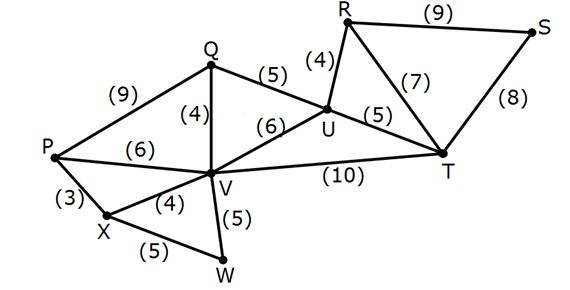Note: In the route shown, the values in brackets denote the distance in kilometres.

(1) A bus does not visit the same city more than once.

(2) Between any two cities only one mode of transport is available, i.e. the new bus.

9) Which of the following might be the minimum bus charge to travel from P to R?

a) Rs. 58

b) Rs. 102

c) 92

d) Rs.78

e) None of these

10) If all the routes connected to City U are blocked, then find the second maximum charge to travel from S to P?

a)Rs. 157

b) 177

c) Rs.168

d) Rs.198

e) None of these

## Click Here for SBI PO Pre 2019 High-Quality Mocks Exactly on SBI Standard

Directions (1-4):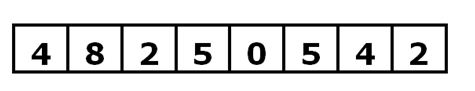(iii) The Number formed by 7th and 8th digits is a product of two consecutive natural numbers.

(vi)The Numbers formed by 7th and 8th digits is six less than the number formed by 1st and 2nd digit.

Products of two consecutive natural numbers are 02, 06, 12, 20, 30, 42, 56, 72 and 90.

From the above two statements we get the following possibilities,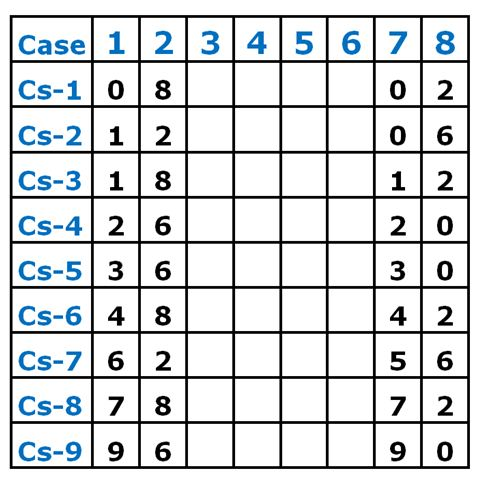(ii) 8th and 1st digits are an even number.

From this statement, we can eliminate Cs-1, Cs-2,Cs-3,Cs-4,Cs-5,Cs-8 and Cs-9.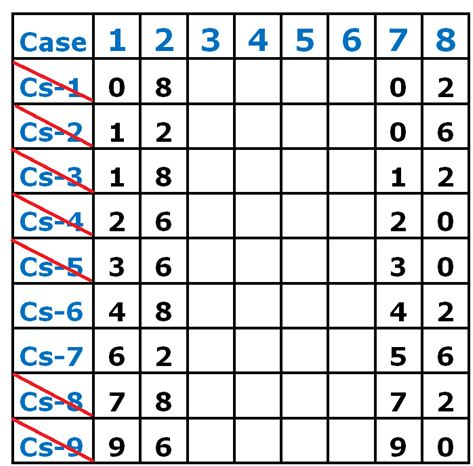(iv) Sum of the two-digit numbers formed by 7rd and 8th digits, and 1st and 2nd digits is a product of two consecutive natural numbers.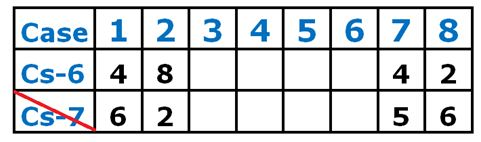Products of two consecutive natural numbers are 02, 06, 12, 20, 30, 42, 56, 72, 90, 110, 132 and 156.

Here we get,

48+42 = 90, this is a product of consecutive number.

62+56 = 118, this is not a product of consecutive number.

So, we can conclude the 1st, 2nd, 7th and 8th digit of the roll number.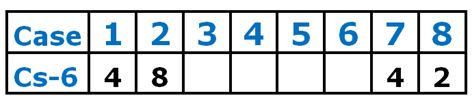(i) The Two digit number formed by 3rd and 4th digits respectively is square of the number formed by 5th and 6th digits.

The square of a number cannot be more than 81 since the square value should be only two digits. Also, zero doesn’t come more than once in the number we can directly omit the squares of 1 to 3.

We get the following possibilities,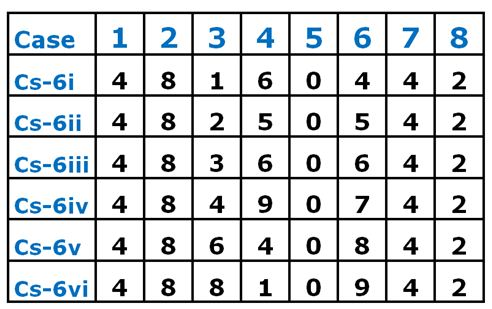(iv) The Two digit number formed by 4th and 7th digits respectively is not a perfect square.

From this statement, Cs-6i and Cs-6iii gets eliminated as the 4th and 7th digit is 64 which is a square number.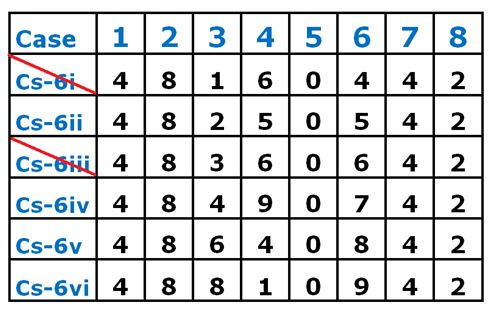(vii) In the number, three digits have been used two times while other two digits have been used only once.

From the above statements,

Only in Cs-6ii we have three digits appeared twice in the number. So, all other case gets eliminated.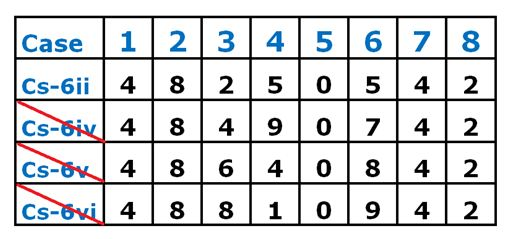So, the Roll number of Mr. Dan Brown is,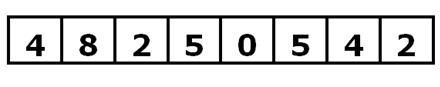Directions (5-8):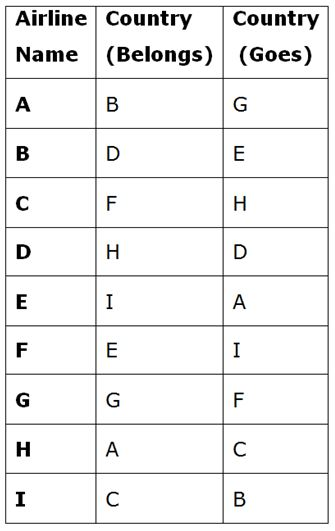(i) Airline-B goes to Country E

(iii) Airline-H belongs to Country A

(v) Airline-D belongs to Country H

(vi) Airline-I belongs to Country C

(viii) Airline-F goes to Country I

(xi) Airline-E goes to Country A

From the above statements we get,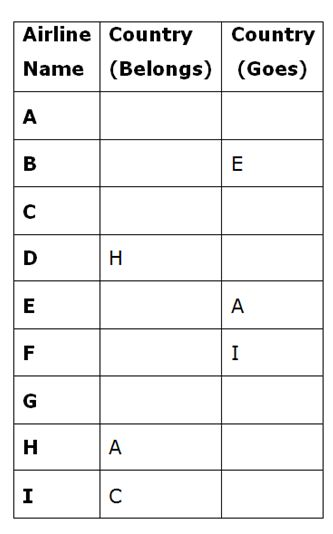(vii) Airline from Country G goes to Country F

(ix) Airline from Country B goes to Country G

(ii) Country H receives airline from Country F

From the above statements we get,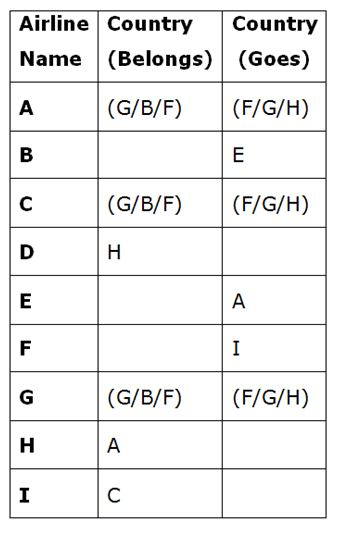From the given information,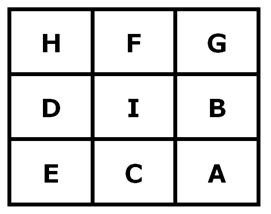We can conclude that,

(1) Since, Airline-E goes to Country A, it should belong to either Country C or I or B. Already we has concluded the Airlines belonging to Country B and Country C. So we can conclude that Airline-E belongs to Country I.

(2) Since, Airline-B goes to Country E, it should belong to either Country D or I or C. Already we has concluded the Airlines belonging to Country I and Country C. So we can conclude that Airline-B belongs to Country D.

(3) Since, only Country E is left we can conclude that Airline-F belongs to Country E.

(4) We could also find the Country where Airline-D goes, which is D. Similarly we could find the Country of the Airlines H and I.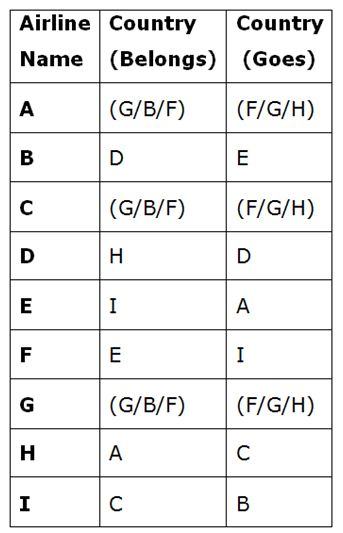(iv) Only one Airline whose name and the alphabet of the country it belongs are same.

(x) Only one Airline whose name and the alphabet of the country it goes are same.

(xii) Airline-A belongs to a country which has Country A as his neighbour.

From the above statements we get,

(1) Since, only one Airline whose name and the country it belongs to are same it could be G.

(2) From, the given information we can conclude that Country B is the neighbour of Country A.So, the Airline-A belongs to Country B.

So, the final arrangement is,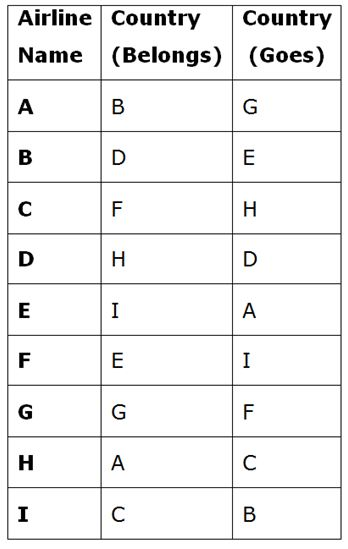Directions (9-10):

The shortest route to travel from P to R is

P – V – U – R and the distance between them is (6+6+4)= 16 km

Charge:

Fixed Charge                              – Rs.9

Service Charge                           – Rs.3

Transport Charge – Rs.5 x 16km   – Rs.80

Total                                           – Rs.92/-

The Possible routes to travel from S to P is

S – T – V – P –>24 km

S – T – V – X – P –> 25 km

S – T – V – W – X – P –> 31 km

S – T – V – Q – P –> 31 km

S – R – T – V – P –> 32 km

S – R – T – V – X – P –> 33 km

S – R – T – V – W – X – P –> 39 km

S – R – T – V – Q – P –> 39 km

Charge:

Fixed Charge                               – Rs.9

Service Charge                            – Rs.3

Transport Charge – Rs.5 x 33 km   – Rs.165

Total                                            – Rs.177/-

## Click Here for SBI PO Pre 2019 High-Quality Mocks Exactly on SBI Standard

Daily Practice Test Schedule | Good Luck

 Topic Daily Publishing Time Daily News Papers & Editorials 8.00 AM Current Affairs Quiz 9.00 AM Current Affairs Quiz (Hindi) 9.30 AM IBPS SO/NIACL AO Prelims – Reasoning 10.00 AM IBPS SO/NIACL AO Prelims – Reasoning (Hindi) 10.30 AM IBPS SO/NIACL AO Prelims – Quantitative Aptitude 11.00 AM IBPS SO/NIACL AO Prelims – Quantitative Aptitude (Hindi) 11.30 AM Vocabulary (Based on The Hindu) 12.00 PM IBPS SO/NIACL AO Prelims – English Language 1.00 PM SSC Practice Questions (Reasoning/Quantitative aptitude) 2.00 PM IBPS Clerk – GK Questions 3.00 PM SSC Practice Questions (English/General Knowledge) 4.00 PM Daily Current Affairs Updates 5.00 PM SBI PO/IBPS Clerk Mains – Reasoning 6.00 PM SBI PO/IBPS Clerk Mains – Quantitative Aptitude 7.00 PM SBI PO/IBPS Clerk Mains – English Language 8.00 PM